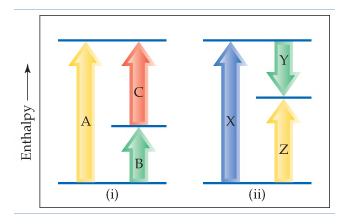# Problem: Consider the two diagrams below.How do both diagram (i) and your equation relate to the fact that enthalpy is a state function?

###### FREE Expert Solution

State function → depends only on the initial and final states of the system, not on how the change occurs

ΔHA is related to ΔHB and ΔHC:

ΔHA = ΔHB + ΔHC###### Problem Details

Consider the two diagrams below.

How do both diagram (i) and your equation relate to the fact that enthalpy is a state function?